Email Us: info@lupinepublishers.comCall Us: +1 (914) 407-6109   57 West 57th Street, 3rd floor, New York - NY 10019, USA

# Lupine Publishers Group

## Lupine Publishers

Submit Manuscript

ISSN: 2644-1217# Biostatistical and Mathematical Analysis on Diversion Cover Plate During Covid-19 Pandemic Volume 4 - Issue 2

Bin Zhao1* and Xia Jiang2

1School of Science, Hubei University of Technology, Wuhan, Hubei, China

2Hospital, Hubei University of Technology, Wuhan, Hubei, China

Received: October 10, 2020   Published: October 20, 2020

*Corresponding author: Dr. Bin Zhao, School of Science, Hubei University of Technology, Wuhan, Hubei, China

DOI: 10.32474/OAJCAM.2022.04.000184

## Abstract

Submarine sailing under the water, because of the attack, misoperation, equipment failure, collision, fire and other factors, the risk of wreck always exists. How to escape and how to carry out effective rescue is a problem that needs to be solved for the navy of submarine owner country since submarine came into being. In order to improve the ability to float out of danger, some submarines are equipped with gas blowing equipment or hydrazine blowing equipment (two kinds of chemical liquid mixed to produce a large amount of high-pressure gas) for emergency ballast jetting, so that the submarine quickly drainage up to float. Some submarines are equipped with quick drift escape device and collective escape with buoyancy ball, buoyancy cylinder, etc. In this paper, the motion characteristics of a simplified diversion cover plate used for buoyancy ball are studied and a differential equation solution is given during COVID-19 pandemic.

Keywords: The submarine; Guide plate; Movement characteristics

## Introduction

As for the release device with the buoyancy ball, the resistance of the submarine in the course of moving is generally borne by the diversion cover plate above it. In case of danger, the diversion cover plate above the buoyancy ball needs to be unlocked by the mechanical locking device and can be opened smoothly and smoothly under the action of buoyancy. Therefore, it is of great significance to study the motion characteristics of fixed axis positive buoyancy diversion cover plate under water during COVID-19 pandemic .

## Method

##### Building A Mechanical Model

There is a uniform rectangular plate completely submerged in water. The length, width and thickness of the rectangular plate are respectively L1 , L2 ,and . Let’s say the h density of the rectangle is pρ and the density of the water is w ρ The rectangular edge of length is L2 articulated to the underwater base, and the rectangular plate is initially fixed horizontally. Now the horizontal fixation of the rectangular plate is removed, and the motion characteristics of the rectangular plate in water need to be solved .

##### Analysis and solutions

There is a dynamic moment formed by gravity and buoyancy for the rectangular plate rotating at a fixed axis in the water (assumed to be Ma ). When the rectangular plate moves in the water, it will be affected by the resistance of water flow and form ar esistance moment (assumed to be MR ). The inertia moment of the rectangular plate is assumed to be Mm . Then, the equation of fixed-axis motion can be expressed as: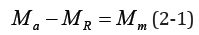Suppose θ(t) is the rotation Angle of the rectangular plate at any time t .

For a uniform rectangular plate, the center of buoyancy coincides with the center of gravity. The dynamic moment formed by buoyancy and gravity can be expressed as: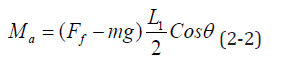Where, Ff is buoyancy, mg is gravity of the rectangular plate, m is mass of the rectangular plate, and g is gravitational acceleration.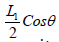is the moment arm of the resultant force of buoyancy and gravity.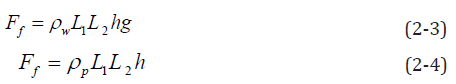Substituting equations (2-3) and (2-4) into equation (2-2) can be obtained:It is assumed that in the process of rotation of the rectangular plate in water, the resistance of water flow is the same at the position equidistant from the rotation center. The resistance moment formed by the resistance of water flow can be expressed as: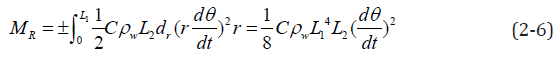Where, is the C water resistance coefficient. Since the resistance of water flow is always opposite to the direction of motion, when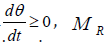takes a positive sign; When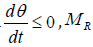takes a negative sign.

The moment of inertia of the rectangular plate can be expressed as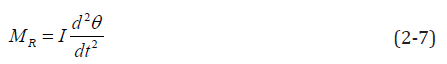Where, I is the rotational inertia of the rectangular plate, which can be expressed as: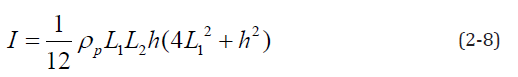Substituting Equation (2-8) into Equation (2-9) can be obtained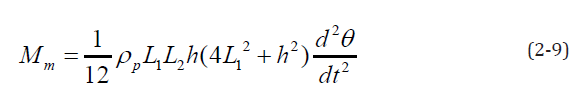Formula (2-5), (2-6) and (2-9) are substituted into formula (2- 1) and can be obtained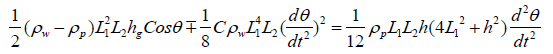The above equation can be simplified to obtain:It can be seen from the above equation that the equation is independent of the width L2 of the rectangular plate, which also means that the motion characteristics of the rectangular plate in water are independent of the width of the rectangular plate. Divide both sides of equation (10) by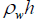, then equation (10) becomesWhen the ratio of plate density to fluid density is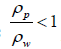, and the initial and angular accelerations are zero, the rectangular plate remains stationary [3-5]. When the ratio of plate density to fluid density is, buoyancy is greater than gravity. If the initial Angle and angular velocity are zero, the rectangular plate moves back and forth periodically, and the vibration amplitude decreases gradually until it reaches zero, and the rectangular plate remains in an upward vertical state. When the ratio of plate density to fluid density is, gravity is greater than buoyancy. If the initial Angle and angular velocity are zero, the rectangular plate moves back and forth periodically, and the vibration amplitude decreases gradually until it reaches zero, and the rectangular plate remains in a downward vertical state.

## Results

The difference method is used to solve the problem. It is assumed that the time interval [0,T] is evenly divided into several time intervals, with a single interval of ΔT. Suppose that t = 0 at time(i) = t1, the initial condition isAssuming that in any interval Δ1T , the rectangular plate moves with uniform acceleration (deceleration), the angular velocity, angle and angular acceleration at time t = (i −1)ΔT can be expressed as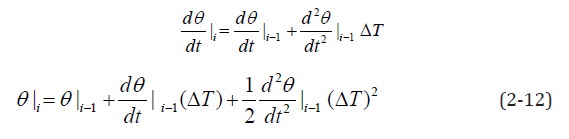## Conflict of Interest

We have no conflict of interests to disclose, and the manuscript has been read and approved by all named authors.

## Acknowledgement

This work was supported by the Philosophical and Social Sciences Research Project of Hubei Education Department (19Y049), and the Staring Research Foundation for the Ph.D. of Hubei University of Technology (BSQD2019054), Hubei Province, China.

Close## Online Submission System

### Submit ManuscriptClick here for Manuscript Sample Templates### Drag and drop files here

or

( For multiple files submission, zip them in a single file to submit. For file zipping software Download )